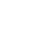×Want to shop in US Dollar? Try our Webshop specifically for North America!

Boots "Sonne"

•••••55.00 EUR
In stock.

Yellow unisex boots with "Comic-Sonne" design. Also available in children's sizes.

• normal fit

• upper material: PU nappa

• sole: TPR

• thin warm lining inside

• zip and lacing

• size: 31-39

UK - EU - US (women) - US (men)

4 = 36 = 6.5 = 5
4.5 = 37 = 7 = 6
5 = 38 = 7.5 = 6
6 = 39 = 8.5 = 7
6.5 = 40 = 9 = 7.5
7.5 = 41 = 10 = 8.5
8 = 42 = 10.5 = 9
9 = 43 = 11.5 = 10
9.5 = 44 = 12 = 10.5
10.5 = 45 = 13 = 11.5
11 = 46 = 13.5 = 12
11.5 = 47 = 14 = 13

RAMMSTEINSHOP RECOMMENDS